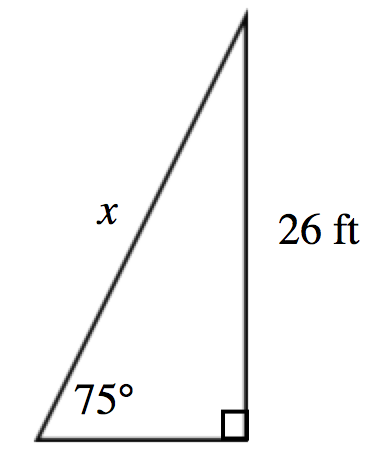### Home > INT2 > Chapter 5 > Lesson 5.1.2 > Problem5-22

5-22.

While shopping at his local home improvement store, Chen notices that the directions for an extension ladder state, “This ladder is most stable when used at a $\mathit{75}^\circ$ angle with the ground.” He wants to buy a ladder that will reach a height of $26$ feet to paint a two-story house. How long does his ladder need to be? Draw a diagram and set up an equation for this situation. Show all work.

$\text{sin}75^{\circ}=\frac{26\;\text{ft}}{x}$

$(x)\text{sin}75^{\circ}=26\;\text{ft}$

$x=\frac{26\; \text{ft}}{\text{sin}75^{\circ}}$

$x\approx 26.9\; \text{feet}$